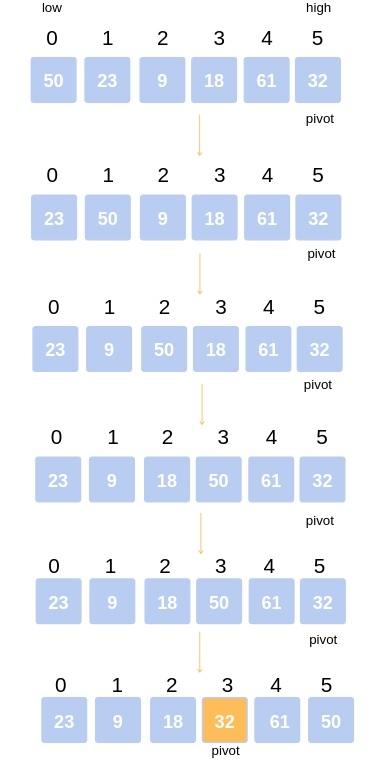# C++ Program for QuickSort?

Quicksort is a sorting technique which uses comparisons to sort an unsorted list( array ). Quicksort is also known as partition exchange sort.

It is not a stable sort, Because the relative order of equal sort items is not preserved. Quicksort can operate on an array, requiring small additional amounts of memory to perform the sorting. It is very similar to selection sort, except that it does not always choose worst-case partition. So, so we can take it as a better formed of selection Sort.

QuickSort is one of the most efficient sorting algorithms and is based on the splitting of an array into smaller ones. The name comes from the fact that quicksort is capable of sorting a list of data elements significantly faster than any of the common sorting algorithms. And like Merge sort, Quick sort also falls into the category of divide and conquer approach of problem-solving methodology.

## Quicksort algorithm works in the following way

• Taking the analogical view in perspective, consider a situation where one had to sort the papers bearing the names of the students, by name. One might use the approach as follows −

• Select a splitting value, say L. The splitting value is also known as Pivot.

• Divide the stack of papers into two. A-L and M-Z. It is not necessary that the piles should be equal.

• Repeat the above two steps with the A-L pile, splitting it into its significant two halves. And M-Z pile, split into its halves. The process is repeated until the piles are small enough to be sorted easily.

• Ultimately, the smaller piles can be placed one on top of the other to produce a fully sorted and ordered set of papers.

• The approach used here is recursion at each split to get to the single-element array.

• At every split, the pile was divided and then the same approach was used for the smaller piles.

• Due to these features, quicksort is also called a partition exchange sort.

Input: arr[] = {7,4,2,6,3,1,5}
Output: 1 2 3 4 5 6 7

## ExplanationAn example might come in handy to understand the concept. Consider the following array: 50, 23, 9, 18, 61, 32

Step 1− Decide any value to be the pivot from the list (generally the last value). Suppose for two values “Low” and “High” corresponding to the first index, and last index.

In our case low is 0 and high is 5.

Values at low and high are 50 and 32 and Value of pivot is 32.

Hence, call for partitioning, rearranging the array in such a way that pivot (32) comes to its actual position. And to the left of the pivot, the array has all the elements less than it, and to the right greater than it.

In the partition function, we start from the first element and compare it with the pivot. Since 50 is greater than 32, we don’t make any change and move on to the next element 23.

Compare again with the pivot. Since 23 is less than 32, we swap 50 and 23. The array becomes 23, 50, 9, 18, 61, 32.

We move on to the next element 9 which is again less than pivot (32) thus swapping it with 50 makes our array as

23, 9, 50, 18, 61, 32.

Similarly, for the next element 18 which is less than 32, the array becomes

23, 9, 18, 50, 61, 32 Now 61 is greater than pivot (32), hence no changes.

Lastly, we swap our pivot with 50 so that it comes to the correct position.

Thus the pivot (32) comes at its actual position and all elements to its left are lesser, and all elements to the right are greater than itself.

Step 2− Hence the array after the first step becomes

23, 9, 18, 32, 61, 50, taking 32 as the pivot.

Step 3− Now the list is divided into two parts:

1. Sublist before pivot: 23, 9, 18

2. Sublist after pivot: 61, 50

Step 4− Repeat the steps for these sublists again.

The final array thus becomes 9, 18, 23, 32, 50, 61.

## Example

#include <stdio.h>
void swap(int *a, int *b) {
int temp;
temp = *a;
*a = *b;
*b = temp;
}
int Partition(int a[], int low, int high) {
int pivot, index, i;
index = low;
pivot = high;
for(i=low; i < high; i++) {
if(a[i] < a[pivot]) {
swap(&a[i], &a[index]);
index++;
}
}
swap(&a[pivot], &a[index]);
return index;
}
int RandomPivotPartition(int a[], int low, int high) {
int pvt, n, temp;
n = rand();
pvt = low + n%(high-low+1);
swap(&a[high], &a[pvt]);
return Partition(a, low, high);
}
int QuickSort(int a[], int low, int high) {
return 0;
}
int main() {
int n, i;
n=7;
int arr[]={7,4,2,6,3,1,5};
int high = n-1;
int low = 0 ;
int pindex;
if(low < high) {
pindex = RandomPivotPartition(arr, low, high);
QuickSort(arr, low, pindex-1);
QuickSort(arr, pindex+1, high);
}
for (i = 0; i < n; i++)
printf("%d ", arr[i]);
return 0;
}